Courses

# Bending Stresses In Beams Civil Engineering (CE) Notes | EduRev

## Civil Engineering (CE) : Bending Stresses In Beams Civil Engineering (CE) Notes | EduRev

The document Bending Stresses In Beams Civil Engineering (CE) Notes | EduRev is a part of the Civil Engineering (CE) Course Civil Engineering SSC JE (Technical).
All you need of Civil Engineering (CE) at this link: Civil Engineering (CE)

Bending Stresses In Beams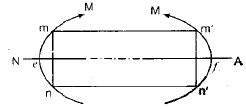THEORY OF PURE BENDING
Following results may be drawn form the observation of pure  bending:

• Two sections mn & m'n' which are parallel to each other before bending rotate through an angle q .

However they remain straight after the deformation.
This means plane section before bending remains plane after bending.

• The  fibre m-m shortens while fibre n-n elongates and there exists no change at the level of e-f which is called neutral axis (N.A.). It always passes through the centroid of the  area. The shortened fibre above N.A. is under compression & elongated fibre is under tension
• From the experimental results it is observed that strain of a fibre is proportional to its distance form the neutral axis.

Let s be the longitudinal stress produced by bending then we have,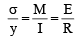Assumptions  made in the theory of simple bending:
(i) The beam is initially straight.
(ii) The beam has constant cross sectional area, with an axis of symmetry. the axis of symmetry is vertical.
(iii) The material is homogeneous  & isotropic
(iv) Hooke's law is valid.
(v) Plane transverse section remains plane after bending.
(vi) Every layer of material is  free to expand or contract longitudinally and laterally under stress and do not exert pressure upon each other. Thus the poison's effect and the interface of the adjoining differently stressed fibre are ignored.
(vii)The value  of Young's modulus (E) for the material is the same in tension and in compression Standard result to be noted:

• For a given stress, the ratio of moments of resistance of a square beam when section is placed with two sides horizontal to that when  the beam is placed with a diagonal horizontal is √2.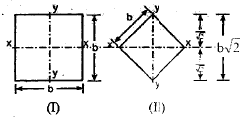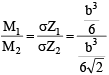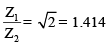Hence arrangement (I) is 41.4% more strong than (II)

• The relation between width 'b' and depth 'd' of the strongest beam that can be cut out of a circular log of diameter 'D' is d

b=d/√2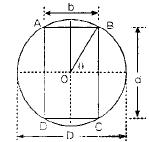For maximum strength, Z should be maximum
Hence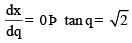b=d/√3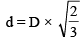b=d/√2

• Three beams have the same length, same allowable bending stress and are subjected to the same maximum bending moment. The cross section of the beams are a circle, a square and rectangle with depth twice the width. Find the ratio of the weights of the circular and rectangular beams with  respect to the square beams.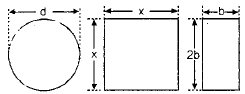Let the circular section be of diameter d Let the square section be of side x Let the rectangular section be of width b & depth 2b.
Since M = s Z Hence, for same bending moments under same bending stresses, the  section modulus should be equal for all three beams.
Hence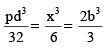Þ d = 1.193 x
b = 0.6299 x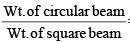=1.118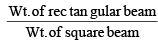= 1.118

• Hence for same strength under same stresses weight of rectangular section is minimum & Weight of circular section is maximum Wrectangular < Wsquare < Wcircular
• A horizontal beam subjected to pure bending is of square section with a diagonal vertical the beam carries a bending moment in vertical plane through the vertical diagonal. it can be shown that by cutting off the top and bottom corners shown shaded in figure, the section modulus  can be increased.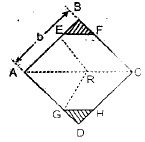Following points may  be noted with reference to above problem.

(i) For maximum strength (Zmax), The portion EB to be  cutoff is given by

EB = (b/9)

(ii) Increase in the section modulus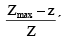100% 5.35%

(i) For a circular section it is possible to increase the section modulus by 0.7% by cutting the segmental portions shaded. (ii) The depth of segment d should be 0.011 times the diameter of the section.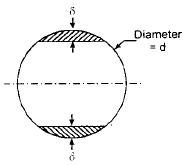FLITCHED  BEAMS
Flitched beam is a composite section consisting of a wooden beam strengthened by metal plates. The arrangement is so connected that the components act together as one beam.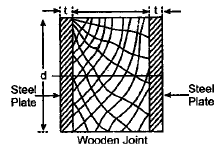The modular ratio m =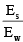MOMENT OF  RESISTANCE OF THE SECTION:

Case– 1 : Flitched are attached symmetrically at sides: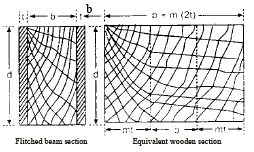Let Mr be the moment of resistance of the section.
Let Mw & Ms be the moments of resistance of wood & steel sections respectively.
Let wooden joint be b unit wide & d unit deep.
Let each steel plate be t units thick and d units deep.
Let σw & σs be the extreme stresses in wood & steel respectively..
Mr = Mw + Ms

Hence the moment of  resistance of the section is the same as that of a wooden member of breadth b + m (2t) and depth d. The rectangular section b + m (2t) units wide and d units deep is called the equivalent wooden section.

Case - 2 : Flitches are attached symmetrically at the top and bottom.
In this case the moment carrying capacity is greater than the moment carrying capacity of side flitches.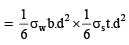But σs = m.σw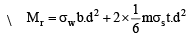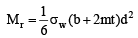BEAMS OF UNIFORM STRENGTH

For economical design, the section of the beam may be reduced towards the support a since bending moment decrease towards the supports. The beam may be designed such that at  every section, the extreme  fibre stress reaches the permissible stress. A beam so designed is called a beam of uniform strength. There are three possible cases for such design. (1) Varying  width by keeping constant depth.
(2) Varying depth by keeping constant width.
(3) Varying width & depth both.

Case-1 : Beam of uniform strength - Constant depth Let the permissible stress be s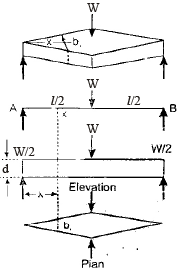• The width of the beam at x is given by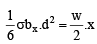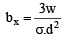• The width of the beam at mid span is given by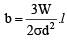Case- 2: Beam of Uniform strength -Constant width.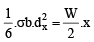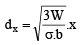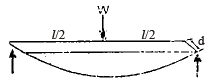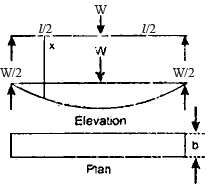Offer running on EduRev: Apply code STAYHOME200 to get INR 200 off on our premium plan EduRev Infinity!

## Civil Engineering SSC JE (Technical)

113 docs|50 tests

,

,

,

,

,

,

,

,

,

,

,

,

,

,

,

,

,

,

,

,

,

;